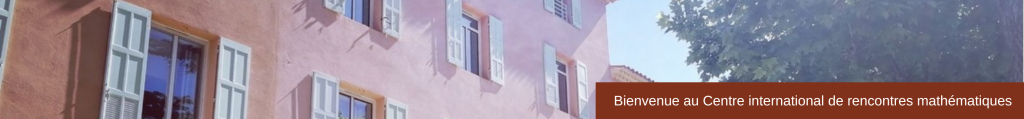## SLIDESProcessusFebruary 15-19, 2016

Stationary increments harmonizable stable fields: upper estimates on path behavior (pdf)

Asymptotic behavior of the Laplacian quasi-maximum likelihood estimator of affine causal processes (pdf)

Overfitting of the Hurst index for a multi fractional Brownian motion (pdf)

Large scale reduction principle and application to hypothesis testing (pdf)

Behavior of the Wasserstein distance between the empirical and the marginal distributions of alpha-dependent sequences (pdf)

Detecting long-range dependence in non-stationary time series (pdf)

Semi-parametric dynamic factor models for non-stationary time series (pdf)

Hölderian weak invariance principle for strictly stationary sequences (pdf)

Quasi-MLE for quadratic ARCH model with long memory​ (pdf)

Statistical inference for bifurcating Markov (pdf)

Mallows’ Quasi-Likelihood Estimation for Log-linear Poisson Autoregressions (pdf)

Phantom distribution functions for dependent random vectors (pdf)

Testing for parameter change in a general class of integer-valued time series models (pdf)

Segmentation of time-series with various types of dependence (pdf)

Strong approximation for additive functionals of geometrically ergodic Markov chains (pdf)

A test for local white noise (and the absence of aliasing) in locally stationary wavelet time series (pdf)

Periodogram Based Tests of Stationarity (pdf)

Adaptive bandwidth selection with cross validation for locallystationary processes (pdf)

Posterior consistency for partially-observed Markov models (pdf)

Fonctional limit theorems for weakly dependent regularly vary-ing time series​​ (pdf)

Fourier based methods for spatial data observed on irregularly spaced locations (pdf)

Detecting a changed segment in a sample (pdf)

Some further properties and applications  of local Gaussian approximation (pdf)

Parameter stability and semiparametric inference in time-varyingARCH processes (pdf)

Classification of nonparametric time trends​ (pdf)

Limit theorems for random fields (pdf)

Time-frequency analysis of locally stationary Hawkes processes (pdf)